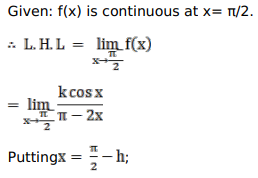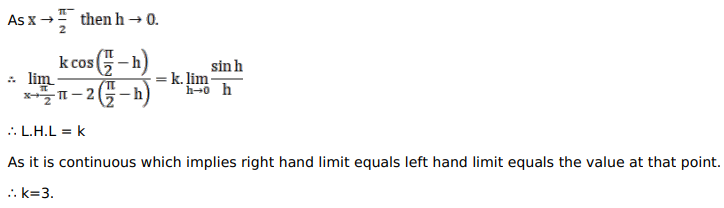# Solve this following

Question:

Mark $(\sqrt{)}$ against the correct answer in the following:

If the function $f(x)=\left\{\begin{array}{c}\frac{k \cos x}{(\pi-2 x)}, \text { when } x \neq \frac{\pi}{2} \\ 3, \text { when } x=\frac{\pi}{2}\end{array}\right.$ be continuous at $x=\frac{\pi}{2}$, then the value of $k$ is

A. 3

B. $-3$

C. $-5$

D. 6

Solution: